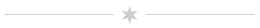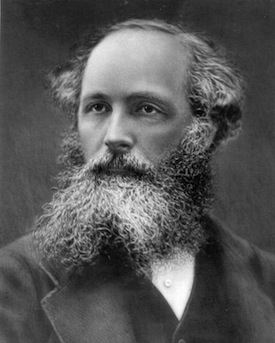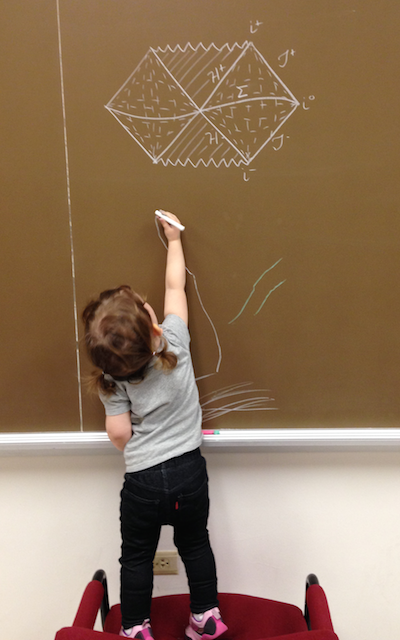# electrodynamics"I have also a paper afloat, with an electromagnetic theory of light, which, till I am convinced of the contrary, I hold to be great guns."

Welcome to Physics 351! In this class we will study charges, currents, electric and magnetic fields, and their interactions. Much of the physics is expressed in a single, remarkable set of equations

\begin{gather} \vec{\nabla} \cdot \vec{E} = \frac{1}{\epsilon_{0}} \rho \vphantom{\frac{\partial\vec{B}}{\partial t}} \\ \vec{\nabla} \times \vec{E} + \frac{\partial\,\vec{B}}{\partial \,t} = 0 \\ \vec{\nabla} \cdot \vec{B} = 0 \vphantom{\frac{\partial\vec{B}}{\partial t}}\\ \vec{\nabla} \times \vec{B} - \mu_{0}\,\epsilon_{0}\,\frac{\partial\,\vec{E}}{\partial\,t} = \mu_{0}\,\vec{J} \end{gather}

This formulation of electromagnetism is due primarily to the Scottish physicist James Clerk Maxwell. His equations, in one form or another, describe phenomenon ranging from the propagation of light to the deflection of a compass needle by a magnetic field.James Clerk Maxwell (1831-1879)

The impact of Maxwell's equations extends well beyond electromagnetism: the Theory of Special Relativity is secreted away inside them, and they are the prototype for a unified description of the basic forces of Nature.

## Fall 2022 Schedule

We will cover most of the first seven chapters of the textbook, along with parts of chapters 8 and 9. The table below is an estimate of how we'll spend our time.

Week Dates Chapter
1 August 30; September 1, 3 1
2 September 6, 8, 10 Labor Day; 1, 2
3 September 13, 16, 18 2
4 September 20, 23, 25 2
5 September 27, 29; October 1 2, 3
6 October 4, 6, 8 3, Exam 1
7 October 11, 13, 15 Fall Break; 3
8 October 18, 20, 22 3, 4
9 October 25, 27, 29 4, 5
10 November 1, 3, 5 5
11 November 8, 10, 12 5, 6
12 November 15, 17, 19 6, Exam 2
13 November 22, 24, 26 7; Thanksgiving
14 November 29; December 1, 3 7, 8
15 December 6, 8, 10 9
16 December 18 Final Exam (1-3pm)

Please keep in mind that these dates are subject to change. We are coming back from remote learning (and who knows what will happen going forward) so there will probably be an adjustment period as we get used to being in-person again. I may move some things around as a result, or I might decide to spend more or less time on a particular topic. I will always notify you about any changes I make to this schedule.

## Assignments

Homework is assigned each week (except for exam weeks) and collected the following week. With the exception of the first few assignments it will usually be due on Monday at the beginning of class. That way you can ask questions during our Friday discussions.

Only some of the problems from each assignment will be graded. I won't tell you which ones, so you need to complete all the problems. We will talk more about how this works in class. Current and past assignments are listed below. Solutions are available for some (not all) problems, but they are not available for download. Please stop by my office if you'd like to see the solutions for a particular assignment.

Assignment 12
The Poynting Vector, and Electromagnetic Waves
Due on Friday, December 9.

The last homework covers a few topics from chapters 8 (the Poynting vector) and 9 (electromagnetic waves). Solutions will be available after you turn it in on Friday, for review before the final exam.

Assignment 11
The Vector Potential, Magnetization
Due on Wednesday, November 30

This assignment covers calculations of the vector potential (including the multipole expansion), and the properties of materials with a permanent magnetization.

Assignment 10
Magnetostatics, Biot-Savart, and Ampère's Law
Due on Monday, November 14

This is a chance to practice calculating the magnetic field using the Biot-Savart law and Ampère's Law.

Assignment 9
Electric Fields in Matter
Due on Monday, November 7

This assignment covers dipoles, polarization, and the response of linear dielectric materials to electric fields..

Assignment 8
The Multipole Expansion
Due on Monday, November 1

This is the last assignment for Chapter 3, covering the sections on the Multipole Expansion of the potential.

Assignment 7
Separation of Variables
Due on Monday, October 24

Separation of variables is a powerful technique for solving the Laplace and Poisson equations. It is often dramatically easier than evaluating the integrals for $$V$$ and $$\vec{E}$$.

Assignment 6
Method of Images
Due on October 17

Using the “method of images” to determine the electric field due to charges near an ideal conductor.

Assignment 5
Electrostatic Potential Energy
Due on Monday, October 3

Homework 5 covers work and potential energy in electrostatics. There is also a bonus problem that investigates some common features of $$1/r^{2}$$ forces. That one won't be graded or checked for completion, but it's an interesting exercise!

Assignment 4
Electrostatic Potential
Due on Monday, September 26

This is the second homework for Chapter 2, covering the electrostatic potential, Gauss's Law, and the behavior of the electric field at surfaces where there is a charge density.

Assignment 3
Electrostatics
Due on Monday, September 19

This is the first homework for Chapter 2, covering Coulomb integrals and Gauss's Law. The rules about using Mathematica and similar tools are stated at the top of the assignment. (They are not allowed, just like on the last assignment.) Click here for some additional tips on problems 5 and 6. Some of the integrals you need are discussed in "A Few Useful Integrals," in the Notes section.

Assignment 2
More Vector Analysis
Due on Wednesday, September 7

This assignment covers the rest of our Math Methods review. Read the instructions at the top of the page – Mathematica and similar tools are not allowed!

Assignment 1
Review of Vector Analysis
Due on Wednesday, August 31

This assignment is due at the beginning of the second class. It is a review to get you up-to-speed on some aspects of vector analysis that we will frequently use in class.

Working with your classmates on these assignments is encouraged! But you should only hand in work that you've completed on your own. If your solution looks just like someone else's work then you need to go back and redo it from scratch. If you can't explain each step of your solution then you haven't completed the problem on your own. Remember: the only way to be ready for the exams is to do the homework yourself.

Warning

Never, ever hand in an assignment that has been copied from a solutions manual. You won't learn anything that way, and it will earn you a grade of zero for that assignment. If it happens more than once it will be reported to the Department Chair and the Dean. Consider yourself warned. Click here to see the College of Arts and Sciences Statement on Academic Integrity.

Grades in the course are primarily determined by homework assignments and exams. The weekly homework grades contribute 35% of your final grade in the class, and two exams (on October 8 and November 19) count 15% each. A cumulative final on Saturday, December 18 (from 1:0-3:00 PM) is worth 30%. The remaining 5% depends on attendance and participation. Asking questions, taking advantage of office hours, and attending both lectures and discussion sections will earn you the full 5%. Check the pdf syllabus for more details.

## References

The main text for the class is Introduction to Electrodynamics (4th Ed) by Griffiths. The tone of the book is casual and most students find it very accessible. When I was an undergraduate I used the the books by Wangsness and Purcell. Those texts might be useful if something in Griffiths isn't clear. A more advanced treatment is given in Jackson's Classical Electrodynamics, which is the text for practically every graduate E&M course.

1. Introduction to Electrodynamics
David J. Griffiths
2. Electromagnetic Fields
Roald K. Wangsness
3. Electricity and Magnetism
Edward M. Purcell
4. Classical Electrodynamics
J.D. Jackson

Griffiths' book has a very complete (for our purposes) discussion of vector calculus as it is used to describe electricity and magnetism. If you'd like to see additional discussions of this material, I recommend the math methods book by Boas, and also the book by Riley, Hobson, and Bence. For a more advanced treatment refer to Arfken and Weber.

1. Mathematical Methods in the Physical Sciences
Mary L. Boas
2. Mathematical Methods for Physics and Engineering
K.F. Riley, M.P. Hobson, and S.J. Bence
3. Mathematical Methods for Physicists
George Arfken and Hans Weber

The Feynman Lectures on Physics, which include a few nice discussions about some of the things we'll talk about in class, are available online. There should also be a copy of the lectures in Isaac & Al's.

From time to time I may supplement the material from the book with my own notes, which will be posted below.

## Lecture Notes

The full set of lecture notes is available on Sakai, organized in the "Lecture Notes" folder under the "Resources" tab. Click here to access the notes.

## Notes

Ever wonder what the $$\vec{E}$$ and $$\vec{B}$$ fields produced by a moving charge look like? This (dense) set of notes solves Maxwell's equations — rewritten in terms of the potentials $$V$$ and $$\vec{A}$$ — for a moving point charge.

Over the course of the semester I've been pretty strict about when you can and cannot use Mathematica. For the most part you've used it to evaluate integrals, or to take care of basic (though tedious) vector calculus operations. To get an idea of what Mathematica can really do, check out the following links:

These blog posts by Michael Trott (a Senior Researcher at Wolfram) explore a wide range of problems in electrostatics and magnetostatics. Trott uses Mathematica – really uses it – to perform calculations and produce visualizations that would take us days or weeks using pencil and paper. If you have Mathematica installed you can download the articles and play around with the various calculations. Even if you don't have Mathematica on your computer, you can still download Wolfram's CDF Player to view interactive results in a browser.

The link in the title will take you to the arXiv page for the article “Introduction to Magnetic Monopoles”, by Dr. Arttu Rajantie. In class we stated that magnetic monopoles don't seem to exist in nature. If you're curious about that statement, this article may be of interest to you. Dr. Rajantie is a Reader in Theoretical Physics at Imperial College in London (the academic rank of “Reader” at a British university is roughly equivalent to “Professor” at an American university).

Two observers in different frames of reference will give different descriptions of charge and current densities. As we saw in class, this means they will also give different descriptions of the electric and magnetic fields produced by these sources. How does this work?

If all you know about is electrostatics and special relativity, you encounter paradoxes that can only be resolved by introducing a new field that exerts a force on moving charges.

A few comments about linear dielectrics, with an example of how to use Gauss's Law to find $$\vec{D}$$ and then relate it to $$\vec{E}$$ using $$\vec{D} = \epsilon_{0} \vec{E} + \vec{P}$$.

Forces between multipoles look much different than the familiar Coulomb force between two point charges — they decrease more rapidly with distance and depend on the multipoles' relative orientations. In these notes we use what we know about the potential energy of pairs of charges and dipoles to work out monopole-dipole and dipole-dipole forces.

A quick review of the Multipole Expansion, with a few example calculations.

Coulomb integrals are hard to evaluate unless everything is very symmetric. However, we can combine them with what we've learned about separation of variables to produce a complete description of the potential in situations where we may not be able to fully evaluate the Coulomb integral itself. These notes explain the technique and give a few examples, including an (infinite Legendre series) expression for the potential due to a charged disk.

These notes provide a detailed discussion of an example we worked out in class: the potential inside and outside a spherical shell with the azimuthally symmetric surface charge density $$\sigma(\theta) = \sigma_{0} \cos\theta$$. Please take a look, especially if you have questions about Assignment 7.

A quick derivation of the result we talked about in class, showing why part of the electric field has a discontinuity at a charged surface.

One of the problems on Assignment 4 leads to an integral of the form \begin{gather} \int dx\,\sqrt{x^2 + \alpha^2} ~. \end{gather} Evaluating this integral requires the application of several different integration techniques, including changes of variables, trig substitutions, and the method of partial fractions.

These notes review two calculations of the electrostatic potential: one obtained by integrating $$d\vec{l} \cdot \vec{E}$$ for a known electric field, and the other by adding up the infinitesimal contributions \begin{gather} dV(\vec{r}, \vec{r}\,') = \frac{dq(\,\vec{r}\,')}{|\,\vec{r}-\vec{r}\,'|} \end{gather} to the potential from each bit of a distribution of charge.

These notes explain why the electric field has a scalar potential, and how to find it based on the distribution of charge. Please familiarize yourself with the results in these notes before Dr. Tangarife's lecture on Friday, September 14.

When a charge distribution is very symmetric, Gauss's Law can help us determine the electric field without having to set up and evaluate Coulomb integrals. These notes briefly review Gauss's Law, Gaussian surfaces, and how to find the electric field for a spherically symmetric distribution of charge.

There were some issues on homework 2 with how to evaluate integrals containing three-dimensional Dirac deltas. Here are a few examples you can work through if you'd like a little more review.

There is an integral requiring a trig substitution that shows up a few places on Homework 3. If you are stuck you should read these notes for an explanation.

Some extra discussion of charge distributions, the transition from a collection of point charges to an infinite number of infinitesimal charges, and the Coulomb integrals for the electric field produced by line, surface, and volume charge densities.

These notes give a brief overview of the Helmholtz theory of vectors, and some important facts about vectors with vanishing divergence or curl. A more complete discussion is given in Appendix B of the text. Some of these ideas will be developed more fully in later chapters.

A quick review of a few integrals that show up again and again on the homework.

The Dirac delta can be a little tricky, so here are some notes that expand on our quick review in class.

A very quick and very rough review of line, surface, and volume integrals with several examples. The part on volume integrals isn't finished, but the stuff on line and surface integrals is there.

A review of orthogonal coordinate systems and vector calculus for students who did not take Phys 301 (Math Methods) in the Spring 2020 semester.

This is a very basic review of line integrals – what they are, how to evaluate them, etc. It may be useful if you're a little rusty on this topic. The file is big (about 22 MB) because of the various plots. Let me know if you find any typos or mistakes and I will post a corrected version.

## E&M Stress Relief

Sometimes the E&M wears you out, and you need a picture of an adorable little kid doing physics to get you back on track. Not a problem.